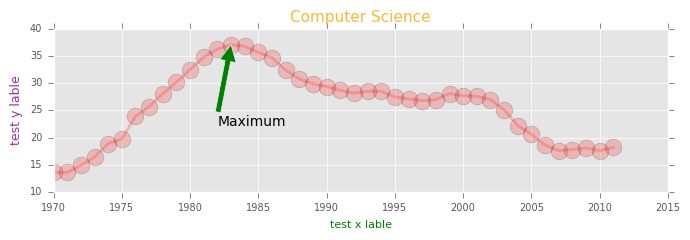Visualization with Matplotlib -1 basics

Customizing plots

subplot

layout

In :
import numpy as np
import matplotlib.pyplot as plt
%matplotlib inline

data set

records of undergraduate degrees awarded to women in a variety of fields from 1970 to 2011

• physical_sciences (representing the percentage of Physical Sciences degrees awarded to women each in corresponding year)
• computer_science (representing the percentage of Computer Science degrees awarded to women in each corresponding year)
In :
year=np.arange(1970,2012)
In :
physical_sciences = np.array([ 13.8,  14.9,  14.8,  16.5,  18.2,  19.1,  20. ,  21.3,  22.5,
23.7,  24.6,  25.7,  27.3,  27.6,  28. ,  27.5,  28.4,  30.4,
29.7,  31.3,  31.6,  32.6,  32.6,  33.6,  34.8,  35.9,  37.3,
38.3,  39.7,  40.2,  41. ,  42.2,  41.1,  41.7,  42.1,  41.6,
40.8,  40.7,  40.7,  40.7,  40.2,  40.1])
In :
computer_science = np.array([ 13.6,  13.6,  14.9,  16.4,  18.9,  19.8,  23.9,  25.7,  28.1,
30.2,  32.5,  34.8,  36.3,  37.1,  36.8,  35.7,  34.7,  32.4,
30.8,  29.9,  29.4,  28.7,  28.2,  28.5,  28.5,  27.5,  27.1,
26.8,  27. ,  28.1,  27.7,  27.6,  27. ,  25.1,  22.2,  20.6,
18.6,  17.6,  17.8,  18.1,  17.6,  18.2])
In :
plt.figure(figsize=[6,3])
# Plot in blue the % of degrees awarded to women in the Physical Sciences
plt.plot(year, physical_sciences, color='blue')

# Plot in red the % of degrees awarded to women in Computer Science
plt.plot(year, computer_science, color='red')

# Display the plot
plt.show()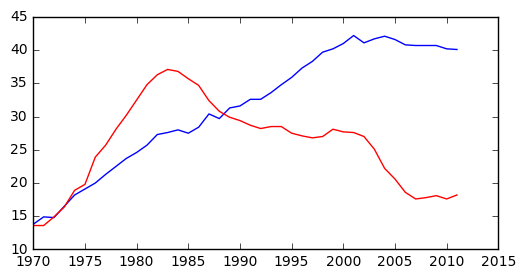Using axes()¶

• In calling plt.axes([xlo, ylo, width, height]), a set of axes is created and made active with lower corner at coordinates (xlo, ylo) of the specified width and height. Note that these coordinates are passed to plt.axes() in the form of a list.
• The coordinates and lengths are values between 0 and 1 representing lengths relative to the dimensions of the figure. After issuing a plt.axes() command, plots generated are put in that set of axes.
In :
plt.figure(figsize=[9,3])

# Create plot axes for the first line plot
plt.axes([0.05,0.05,0.425,0.9])

# Plot in blue the % of degrees awarded to women in the Physical Sciences
plt.plot(year,physical_sciences, color='blue')

# Create plot axes for the second line plot
plt.axes([.525,0.05,0.425,0.9])

# Plot in red the % of degrees awarded to women in Computer Science
plt.plot(year,computer_science, color='red')

# Display the plot
plt.show()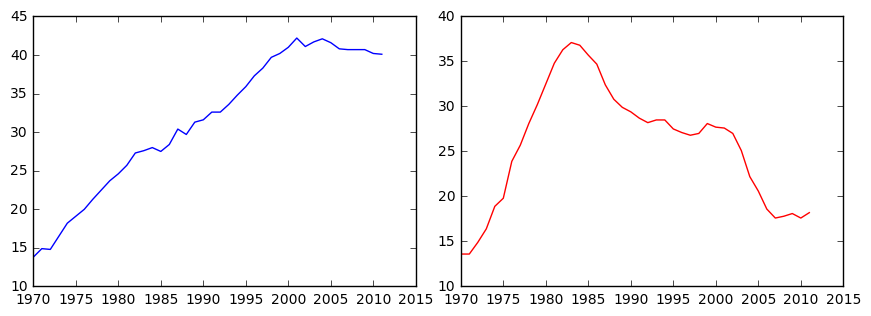Using subplot()¶

• The command plt.axes() requires a lot of effort to use well because the coordinates of the axes need to be set manually. A better alternative is to use plt.subplot() to determine the layout automatically.
• plt.subplot(m, n, k) to make the subplot grid of dimensions m by n and to make the kth subplot active (subplots are numbered starting from 1 row-wise from the top left corner of the subplot grid).
In :
plt.figure(figsize=[9,3])

# Create a figure with 1x2 subplot and make the left subplot active
plt.subplot(1,2,1)

# Plot in blue the % of degrees awarded to women in the Physical Sciences
plt.plot(year, physical_sciences, color='blue')
plt.title('Physical Sciences')

# Make the right subplot active in the current 1x2 subplot grid
plt.subplot(1,2,2)

# Plot in red the % of degrees awarded to women in Computer Science
plt.plot(year, computer_science, color='red')
plt.title('Computer Science')

# Use plt.tight_layout() to improve the spacing between subplots
plt.tight_layout()
plt.show()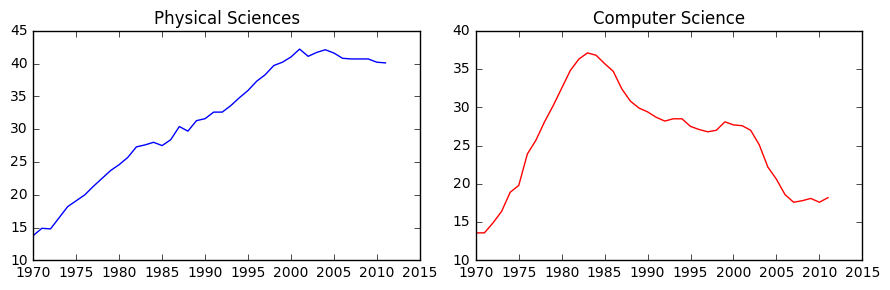health (representing the percentage of Computer Science degrees awarded to women in each corresponding year

education

In :
health = np.array([ 77.1,  75.5,  76.9,  77.4,  77.9,  78.9,  79.2,  80.5,  81.9,
82.3,  83.5,  84.1,  84.4,  84.6,  85.1,  85.3,  85.7,  85.5,
85.2,  84.6,  83.9,  83.5,  83. ,  82.4,  81.8,  81.5,  81.3,
81.9,  82.1,  83.5,  83.5,  85.1,  85.8,  86.5,  86.5,  86. ,
85.9,  85.4,  85.2,  85.1,  85. ,  84.8])
education = np.array([ 77.1,  75.5,  76.9,  77.4,  77.9,  78.9,  79.2,  80.5,  81.9,
82.3,  83.5,  84.1,  84.4,  84.6,  85.1,  85.3,  85.7,  85.5,
85.2,  84.6,  83.9,  83.5,  83. ,  82.4,  81.8,  81.5,  81.3,
81.9,  82.1,  83.5,  83.5,  85.1,  85.8,  86.5,  86.5,  86. ,
85.9,  85.4,  85.2,  85.1,  85. ,  84.8])

2x2 subplot layout¶

In :
# Create a figure with 2x2 subplot layout and make the top left subplot active
plt.subplot(2,2,1)

# Plot in blue the % of degrees awarded to women in the Physical Sciences
plt.plot(year, physical_sciences, color='blue')
plt.title('Physical Sciences')

# Make the top right subplot active in the current 2x2 subplot grid
plt.subplot(2,2,2)

# Plot in red the % of degrees awarded to women in Computer Science
plt.plot(year, computer_science, color='red')
plt.title('Computer Science')

# Make the bottom left subplot active in the current 2x2 subplot grid
plt.subplot(2,2,3)

# Plot in green the % of degrees awarded to women in Health Professions
plt.plot(year, health, color='green')
plt.title('Health Professions')

# Make the bottom right subplot active in the current 2x2 subplot grid
plt.subplot(2,2,4)

# Plot in yellow the % of degrees awarded to women in Education
plt.plot(year, education, color='yellow')
plt.title('Education')

# Improve the spacing between subplots and display them
plt.tight_layout()
plt.show()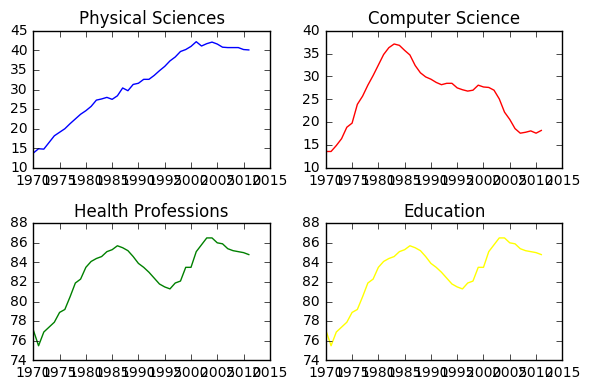Using xlim(), ylim()¶

• set x- and y-limits of plots, e.g. plt.xlim() to set the x-axis range
In :
plt.figure(figsize=[9,3])

# Plot the % of degrees awarded to women in Computer Science and the Physical Sciences
plt.plot(year,computer_science, color='red')
plt.plot(year, physical_sciences, color='blue')

plt.xlabel('Year')
plt.ylabel('Degrees awarded to women (%)')

# Set the x-axis range
plt.xlim([1990,2010])

# Set the y-axis range
plt.ylim([0,50])

# Add a title and display the plot
plt.title('Degrees awarded to women (1990-2010)\nComputer Science (red)\nPhysical Sciences (blue)')
plt.show()

# Save the image as 'xlim_and_ylim.png'
plt.savefig('xlim_and_ylim.png')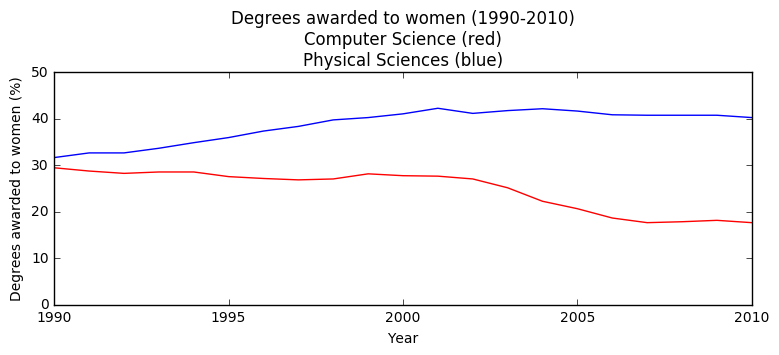<matplotlib.figure.Figure at 0x7f32a7dca850>

Using axis()¶

• alternatively, you can pass a 4-tuple to plt.axis() to set limits for both axes at once.
• save plot using savefig()
In :
plt.figure(figsize=[7,3])

# Plot in blue the % of degrees awarded to women in Computer Science
plt.plot(year,computer_science, color='blue')

# Plot in red the % of degrees awarded to women in the Physical Sciences
plt.plot(year, physical_sciences,color='red')

# Set the x-axis and y-axis limits
plt.axis((1990,2010,0,50))

# Show the figure
plt.show()

# Save the figure as 'axis_limits.png'

plt.savefig('axis_limits.png')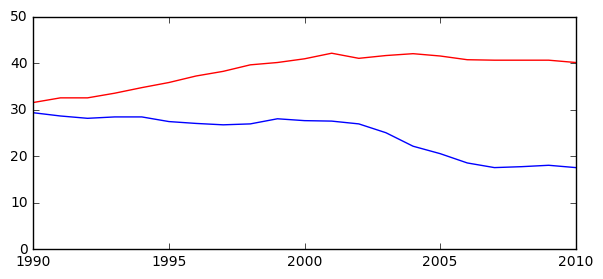<matplotlib.figure.Figure at 0x7f32a7e68e90>

Other axis() options¶

Invocation Result
axis(‘off’) turns off axis lines, labels
axis(‘equal’) equal scaling on x, y axes
axis(‘square’) forces square plot
axis(‘tight’) sets xlim(), ylim() to show all data
In :
plt.figure(figsize=[20,3])
plt.subplot(1,2,1)

# Plot in blue the % of degrees awarded to women in Computer Science
plt.plot(year,computer_science, color='blue')
# Plot in red the % of degrees awarded to women in the Physical Sciences
plt.plot(year, physical_sciences,color='red')
# Set the x-axis and y-axis limits
plt.axis('equal')

plt.subplot(1,2,2)

# Plot in blue the % of degrees awarded to women in Computer Science
plt.plot(year,computer_science, color='blue')
# Plot in red the % of degrees awarded to women in the Physical Sciences
plt.plot(year, physical_sciences,color='red')
# Set the x-axis and y-axis limits
plt.axis('tight')

# Show the figure
plt.show()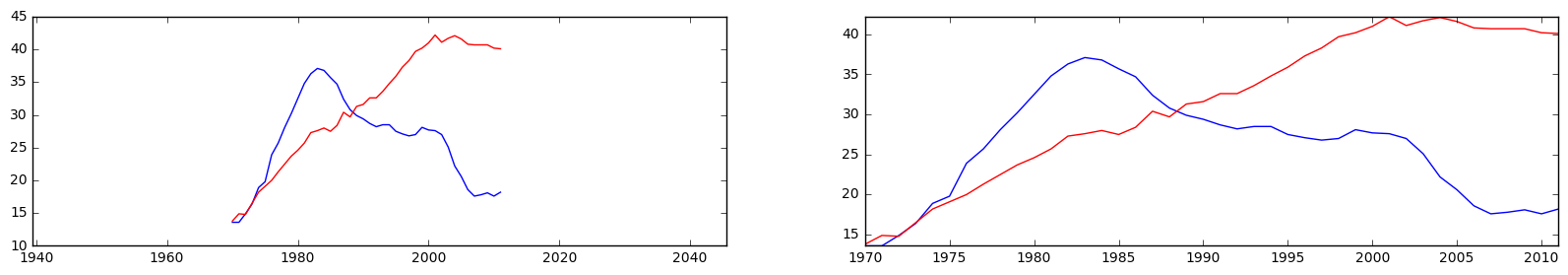Using legend()¶

In :
plt.figure(figsize=[7,2.5])

# Specify the label 'Computer Science'
plt.plot(year, computer_science, color='red', label='Computer Science')

# Specify the label 'Physical Sciences'
plt.plot(year, physical_sciences, color='blue', label='Physical Sciences')

# Add a legend at the lower center
plt.legend(loc='upper right')

# Add axis labels and title
plt.xlabel('Year')
plt.ylabel('Enrollment (%)')
plt.show()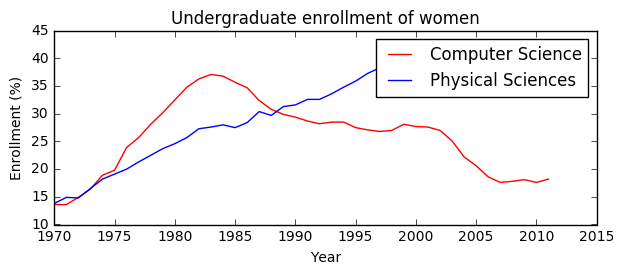Legend locations¶

string code string code string code
'upper left' 2 'upper center' 9 'upper right' 1
'center left' 6 'center' ' 10 'center right' 7
'lower left' 3 'lower center' 8 'lower right' 4
'best' 0 'right' 5

Using annotate()¶

• To enable an arrow, set arrowprops=dict(facecolor='black'). The arrow will point to the location given by xy and the text will appear at the location given by xytext
In :
plt.figure(figsize=[7,2.5])

# Plot with legend as before
plt.plot(year, computer_science, color='red', label='Computer Science')
plt.plot(year, physical_sciences, color='blue', label='Physical Sciences')
plt.legend(loc='lower right')

# Compute the maximum enrollment of women in Computer Science: cs_max
cs_max = computer_science.max()

# Calculate the year in which there was maximum enrollment of women in Computer Science: yr_max
yr_max = year[computer_science.argmax()]

# Add a black arrow annotation
plt.annotate(s='Maximum', xy=(yr_max, cs_max), xytext=(yr_max-30,cs_max+8), arrowprops={'facecolor':'cyan'})

# Add axis labels and title
plt.xlabel('Year')
plt.ylabel('Enrollment (%)')
plt.show()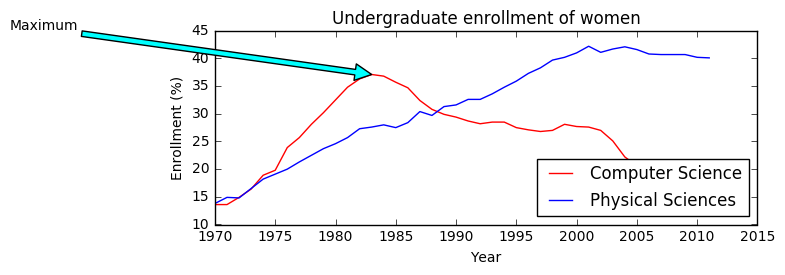Modifying styles¶

• Matplotlib comes with a number of different stylesheets to customize the overall look of different plots. To activate a particular stylesheet you can simply call plt.style.use() with the name of the style sheet you want.
• To list all the available style sheets you can execute: print(plt.style.available)
In :
print(plt.style.available)
[u'seaborn-darkgrid', u'seaborn-notebook', u'classic', u'seaborn-ticks', u'dark_background', u'bmh', u'seaborn-talk', u'grayscale', u'ggplot', u'fivethirtyeight', u'seaborn-colorblind', u'seaborn-deep', u'seaborn-whitegrid', u'seaborn-bright', u'seaborn-poster', u'seaborn-muted', u'seaborn-paper', u'seaborn-white', u'seaborn-pastel', u'seaborn-dark', u'seaborn-dark-palette']

set smaller font of axis¶

In :
plt.figure(figsize=[7,2.5])

# Set the style to 'ggplot'
plt.style.use('ggplot')

# Plot the enrollment % of women in Computer Science
plt.plot(year, computer_science, 'ro-',alpha=.2,linewidth=2, markersize=12)
plt.title('Computer Science',fontsize=11,alpha=.8,color='orange')
plt.xlabel('test x lable',fontsize=8,color='g')
plt.ylabel('test y lable',fontsize=9,color='purple',alpha=.8)

plt.tick_params(labelsize=7)